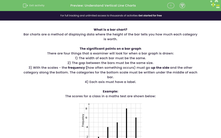# Understand Vertical Line Charts

In this worksheet, students will practise reading vertical line charts.Key stage:  KS 4

Year:  GCSE

GCSE Subjects:   Maths

GCSE Boards:   AQA, Eduqas, Pearson Edexcel, OCR,

Curriculum topic:   Statistics

Curriculum subtopic:   Statistics Interpreting and Representing Data

Difficulty level:#### Worksheet Overview

What is a bar chart?

Bar charts are a method of displaying data where the height of the bar tells you how much each category is worth.

The significant points on a bar graph

There are four things that a examiner will look for when a bar graph is drawn:

1) The width of each bar must be the same.

2) The gap between the bars must be the same size.

3) With the scales - the frequency (how often something occurs) must go up the side and the other category along the bottom. The categories for the bottom scale must be written under the middle of each bar.

4) Each axis must have a label.

Example:

The scores for a class in a maths test are shown below:How many pupils are in the class?

All we have to do for this one is work out what each bar on the chart is worth. This is given by reading off the height on the side.

2 + 4 + 5 + 8 + 6 = 25

Let's have a go at some questions.

### What is EdPlace?

We're your National Curriculum aligned online education content provider helping each child succeed in English, maths and science from year 1 to GCSE. With an EdPlace account you’ll be able to track and measure progress, helping each child achieve their best. We build confidence and attainment by personalising each child’s learning at a level that suits them.

Get started